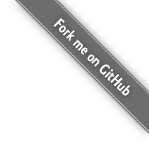# The reflective language Black## Warm up

Just like Scheme... at first.

(+ 2 1) (define inc (lambda (x) (+ x 1))) (inc 2) ((lambda (x) (+ x 1)) 2) (map inc '(1 2 3)) (map (lambda (x) (+ x 1)) '(1 2 3))

(incr 2)

Notice the prompt change, from 0-7 to 1-0. The first number is the meta level, i.e. the n in metan level. The second number is just the iteration counter at this level.

Two ways to go back down.

(1) call old-cont.

(old-cont (lambda (x) (+ x 1)))

(2) use (base-eval exp env cont).

(base-eval 1 '() (lambda (v) v)) incr ;; typo again (base-eval 'inc old-env (lambda (v) v)) incr ;; typo again (base-eval 'inc old-env old-cont)

Notice we needed to quote inc at the meta-level. Why? Let's jump back to the meta1 level and see. We can jump levels normally with exit, no need to fake errors.

(exit 'hello) ;; inc is not defined inc

Oh, we loaded meta2 now! Let's go back to 0:

(old-cont 1) (old-cont 'back-to-user-level)

To recap, a variable is local to a level:

(exit 'hello) (define foo 1) (old-cont foo) ;; foo is unbound at level 0 foo ;; but at level 1, it is defined foo ;; going back again (old-cont foo)

Can we access some meta level without pushing away (or popping? which way does it go?) without leaving the level? Yes, with EM.

(EM foo) (EM (define bar 2)) (EM bar) bar ;; not bound (EM (EM 1)) (EM (EM foo)) ;; oops, we just loaded level 3 (old-cont 1)

Interesting, we got straight back to 0 from 3, some jumping indeed!

## The Mystery of Meta-Continuations Revealed

Each level in the tower has its own environment and continuation.

### Bouncing Up and Down

First, we redefine evaluation of variable (by mutating the meta level) so that when the variable name is _dummy we do something special: we call the continuation first with 1, then 2, then 3.

(EM (begin (define old-eval-var eval-var) (set! eval-var (lambda (e r k) (if (eq? '_dummy e) (begin (k 1) (k 2) (k 3)) (old-eval-var e r k)))) ))

What happens when we evaluate _dummy?

_dummy

OK, so it seems that it just jumps out at the first 1? Not so fast:

_dummy ;; 1 (exit 'up) ;; 2 (exit 'up) ;; 3 (exit 'up) ;; up (meta level)

### Being Pushy... Bug or Feature?

(define where_am_i 'user) (EM (define where_am_i 'meta)) (EM (let ((old-eval-var eval-var) (__k (lambda (x) x))) (set! eval-var (lambda (e r k) (if (eq? e '__k) (k __k) (begin (if (eq? e '_) (set! __k k) '()) (old-eval-var e r k))))))) (define _ 0) (+ _ 1) where_am_i ;; user (EM where_am_i) ;; meta (__k 2) where_am_i ;; user (EM where_am_i) ;; user (!!!) (EM (EM where_am_i)) ;; meta (__k 2) ;; unbound variable __k

If you'd like to learn more about semantics of reflective towers, including pushy vs. jumpy continuations, see the paper Intensions and Extensions in a Reflective Tower (PDF) by Danvy et al.

## Fun with Towers

The loaded code in the examples below is also on Github.

### Counting Evaluations

(exec-at-metalevel (load "examples/instr2.blk")) (load "examples/church.scm") (instr (prd c2)) (instr (prd-alt c2)) (instr (to_int (prd-alt c2))) (instr (to_int (prd c2)))

### There and Back Again

There is a cute trick by Danvy et al. (ICFP, extended journal version) to construct (cnv xs ys) = (zip xs (reverse ys)) in n recursive calls and no auxiliary data list, where xs and ys are lists of size n.

It's fun to think about it, so let's not give it away. Suffice to say that it's helpful to visualize the stack.

(EM (load "examples/utils.blk")) (EM (load "examples/taba.blk")) (load "examples/cnv.scm") (taba (cnv3 walk) (cnv3 '(1 2 3) '(a b c))) (load "examples/pal.scm") (taba (pal_c walk) (pal_c '(1 2 2 1)))
(EM (load "examples/utils.blk")) (EM (load "examples/stack.scm")) (EM (load "examples/taba3.blk")) (load "examples/cnv.scm") (taba (cnv3 walk) (cnv3 '(1 2 3) '(a b c))) (load "examples/pal.scm") (taba (pal_c walk) (pal_c '(1 2 2 1)))